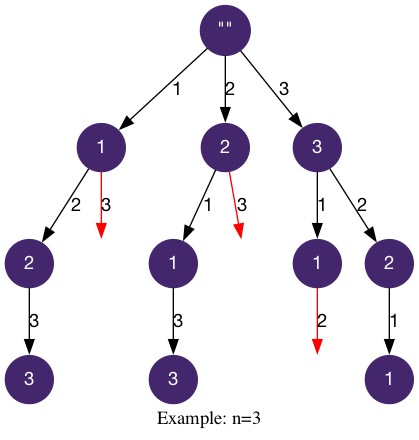# LeetCode Beautiful Arrangement Solution

Suppose you have `n` integers labeled `1` through `n`. A permutation of those `n` integers `perm` (1-indexed) is considered a beautiful arrangement if for every `i` (`1 <= i <= n`), either of the following is true:

• `perm[i]` is divisible by `i`.
• `i` is divisible by `perm[i]`.

Given an integer `n`, return the number of the beautiful arrangements that you can construct.

Example 1:

Input: `n = 2`

Output: `2`

Explanation:

The first beautiful arrangement is `[1,2]`: - `perm = 1` is divisible by `i = 1` - `perm = 2` is divisible by `i = 2` The second beautiful arrangement is `[2,1]`: - `perm = 2` is divisible by `i = 1` - `i = 2` is divisible by `perm = 1`

Example 2:

Input: `n = 1`

Output: `1`

Constraints:

• `1 <= n <= 15`

## Solution

Since this problem asks for the number of beautiful solutions, we will use the aggregation template for backtracking. We will fill in the logic.

• additional state: `unvisited` to store unused numbers.
• `is_leaf`: `i == n+1`, all the `n` numbers are used.
• `get_edges`: all of the numbers from `1` to `n`
• `is_valid`: `not visited[num] and i % num == 0 or num % i == 0`, check whether `num` is unused and `num` is beautiful for index `i`.

#### Implementation

``````1def countArrangement(self, n: int) -> int:
2    visited = [False] * (n+1)
3    def findBeautiful(i):
4        if i == n+1:
5            return 1
6        count = 0
7        for num in range(1, n+1):
8            if (not visited[num]) and (i % num == 0 or num % i == 0):
9                visited[num] = True
10                count += findBeautiful(i + 1)
11                visited[num] = False
12        return count
13    return findBeautiful(1)``````